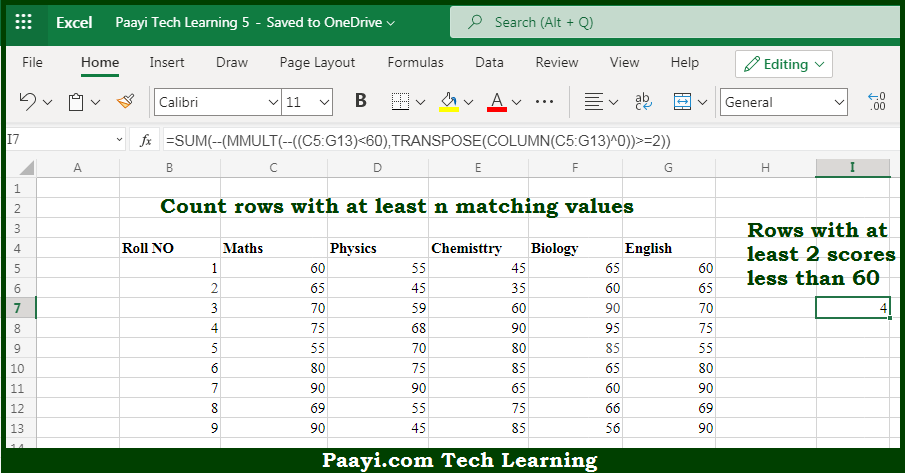# Learn How to Count Rows With At Least n Matching Values in Microsoft Excel

Written by | 0 Comments | 418 Views

In this article, you will learn how to COUNT various things in Microsoft Excel using a single or combination of functions and its purpose in Microsoft Excel. You will also get to know how to Count Rows With At Least n Matching Values & generic formula

Count Rows With At Least n Matching Values in Microsoft Excel

The main purpose of this formula is to count rows that contain specific values. Here we will learn how to count the rows with at least n matching values in Microsoft Excel. That implies, with the help of a formula based on the array and using functions like   MMULT, TRANSPOSE, COLUMN, and SUM you can able to count rows that contain specific values. So, with the help of this formula, you can able to count rows containing n matching values.

General Formula to Count Rows With At Least n Matching Values

{=SUM(--(MMULT(--(criteria),TRANSPOSE(COLUMN(data)^0))>=N))}

The Explanation for the Count Rows With At Least n Matching ValuesSo we know that with the help of the given formula above you can able to count rows that contain specific values. Here we will learn how to count the rows with at least n matching values in Microsoft Excel. As we know that in the example provided, the COLUMN function is used as a way to generate a numeric array of the right size. Al the matrix multiplication needs the column count in array1 7 to equal the row count in array2. So, with the help of this formula, you can able to count the rows with at least n matching values.